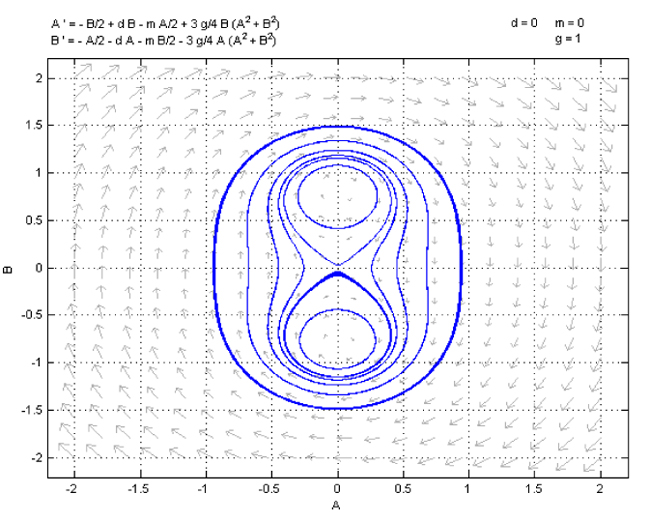Fig. (5) Phase plots of the A1 - B1 dynamics. The left plot shows the dynamics for γ = 1 and the right plot shows the dynamics for γ = -1. Both plots have α = 0 and μ = 0.Back to Article